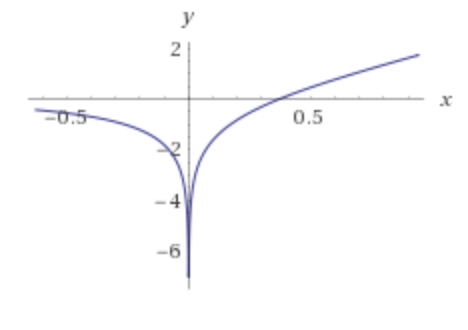##### Page tree
Go to start of banner

# Exponential Integral (Ei or E1)

@wikipedia

Two different functions of real argument $//$ are called this way:

 (1)
 (2)

which are related to each other as:

 (3)

There is a trend to moving from $//$ definition (which was dominating in the past) towards  $//$ which becomes more and more popular nowdays.Fig. 1. A sample graph of $//$

 (4)
 (5)
 (6)
 (7)

# Approximations

$//$

$//$

 (8)
 (9)

where $//$... is Euler–Mascheroni constant

$//$

 (10)

# Application

The real-value positive function $//$ of two real-value positive arguments (time $//$ and radial coordinate $//$):

 (11)

honours a planar axial-symmetric diffusion equation with homogenous initial and boundary conditions

 (12)
 (13)
 (14)
 (15)

and is widely used in radial heat-mass transfer analysis.